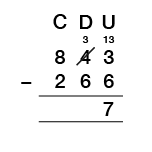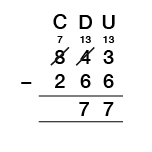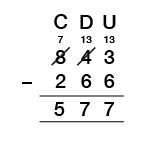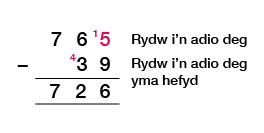Science, Maths & Technology

### Become an OU studentEveryday maths 1 (Wales)

Start this free course now. Just create an account and sign in. Enrol and complete the course for a free statement of participation or digital badge if available.

# 1.6 Add and subtract large numbers

We add large numbers in the same way as we add smaller numbers:

Interactive feature not available in single page view (see it in standard view).

### Activity 6: Adding whole numbers

Complete the following tasks without using a calculator:

1. 8 936 + 453
2. 3 291 + 2 520
3. 35 + 214 + 9 963
4. 28 550 + 865
5. 243 552 + 64 771
6. 698 441 + 323 118

Remember you can check your calculations using the inverse method, which means using the opposite type of sum to check that your answer is correct. For example, you can use subtraction to check that an addition calculation is correct:

630 + 295 = 925 (addition)

925 – 295 = 630 (subtraction to check)

1. 9 389
2. 5 811
3. 10 212
4. 29 415
5. 308 323
6. 1 021 559

## Subtraction

There are different methods that can be used to subtract numbers. You need to find the method that works for you.

## Decomposition method

For example:

843 – 266

1.

• a.Start with the units: subtract 6 from 3. (This can’t be done.)
• b.There are four tens in the tens column. One of these can be given to the units column.
• c.If 10 is added to the original 3 we now have 13 in the units column: 13 – 6 = 7.
• d.7 is placed on the answer line in the units column.2.

• a.Now move on to the tens column: subtract 6 from 3. There are only three tens left, because one 10 was added to the units column. (This can’t be done.)
• b.There are eight hundreds in the hundreds column. Taking one from the hundreds column and moving it to the tens column makes 13 in the tens column: 13 – 6 = 7.
• c.7 is placed on the answer line in the tens column.3.

• a.There is now a 7 in the hundreds column.
• b.Subtract 3 from 7: 7 – 2 = 5.
• c.This is placed on the answer line in the hundreds column.You need to be careful when trying to subtract with zeros; for example, 800 – 427. The following video shows the decomposition method in full, including dealing with zeros:

Interactive feature not available in single page view (see it in standard view).

## ‘Borrow and pay back’ method

For example:

765 – 39

2. You borrow 10 to make it 15.
3. Now you have borrowed, you must ‘pay back’ by adding 1 to the tens column of the number that you are subtracting. This increases both numbers by ten (5 + 10 = 15 and 39 + 10 = 49). The difference stays the same.### Activity 7: Subtracting whole numbers

Complete this activity using the subtraction method that you are most familiar with. Do not use a calculator.

1. 9 965 – 742
2. 8 163 – 7 481
3. 27 364 – 9 583
4. 600 987 – 4 500
5. 975 046 – 74 308
6. 587 342 – 369 453

Remember you can check your calculations using the inverse method, which means using subtraction to check that your answer to an addition calculation is correct. For example:

630 + 295 = 925 (addition)

925 – 295 = 630 (subtraction to check)